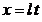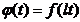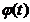§ 2 Generalized Fourier series and Fourier - Bessel series

1. Generalized Fourier Series

If the continuous function is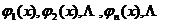( 1 )

Orthogonal on a certain interval , and if the function is absolutely integrable on , then the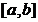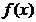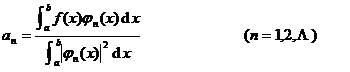is the series of coefficients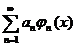is called the generalized Fourier series of functions with respect to the orthogonal function system ( 1 ) , denoted asf ( x ) ~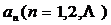are called the Fourier coefficients of the orthogonal function system ( 1 ) .Let ( 1 ) be a standard orthogonal function system, that is, it satisfies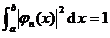then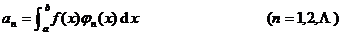At this time, with respect to, there is Bessel's inequality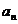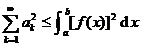(is a square integrable function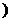If for any square-integrable function, the closedness equation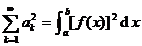It is said that the orthogonal function system at this time is closed .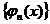2. Fourier - Bessel series

[ Fourier - Bessel series ]

1   Let o be the positive root of the Bessel function (see Chapter 12), then the function system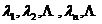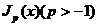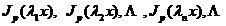Orthogonal by weight x on [0, 1] , i.e.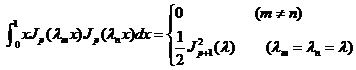2 o  For all functions that are absolutely integrable on [0, 1] , its Fourier - Bessel series can be madef ( x ) ~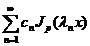in the formula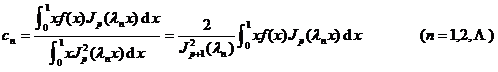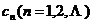are called the Fourier - Bessel coefficients of a function .3 o  If it is continuous everywhere on [0, 1] except for a finite number of discontinuous points of the first kind and is segment-wise differentiable, then its Fourier - Bessel series converges at that time, and at the continuous points, the series The sum equals , at the discontinuity, the series sum equals ;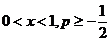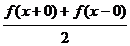If it is absolutely integrable on [0 , 1] , continuous in the interval and has absolutely integrable derivatives, then its Fourier-Bessel series converges uniformly in every interval ;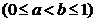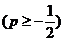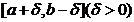If it is absolutely integrable over [0 , 1] , continuous over the interval and has absolutely integrable derivatives, at the same time , then its Fourier - Bessel series converges uniformly over every interval .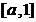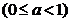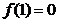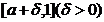[ Fourier - Bessel series of the second kind ]

1 o  Let it be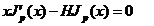( H is a constant)

The positive root of , then, at that time , the function system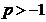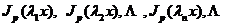Orthogonal by weight x on [0, 1] .

If it is absolutely integrable on [0 , 1] , then its generalized Fourier series with respect to the above orthogonal system is called a Fourier - Bessel series of the second kind , namely~in the formula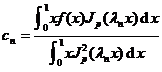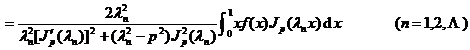2 o  If the function is piecewise differentiable on [0, 1] (with at most a finite number of discontinuities of the first kind), then its Fourier - Bessel series of the second kind converges on 0 < x < 1 , and Equal at continuous points and equal at discontinuous points ;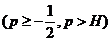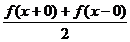If the function is continuous on [0 , 1] , twice differentiable (except for a finite number of points), and = 0, , bounded, then its second kind of Fourier - Bessel series is then , at each The interval [ ,1] (0< <1) is absolutely and uniformly convergent; at the same time , it is absolutely and uniformly converged on the entire interval [0 , 1] .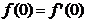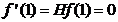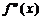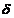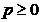[ Fourier - Bessel series over the interval [0 , l ] ]

Suppose it is absolutely integrable on [0 , l ] , then its Fourier - Bessel series isf ( x ) ~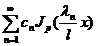in the formula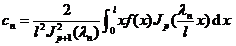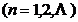For the Fourier - Bessel series of the second kind,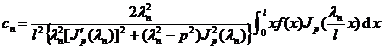Regarding the convergence of the series, we can only discuss the convergence of the corresponding Fourier - Bessel series on [0 , 1] by transforming , .